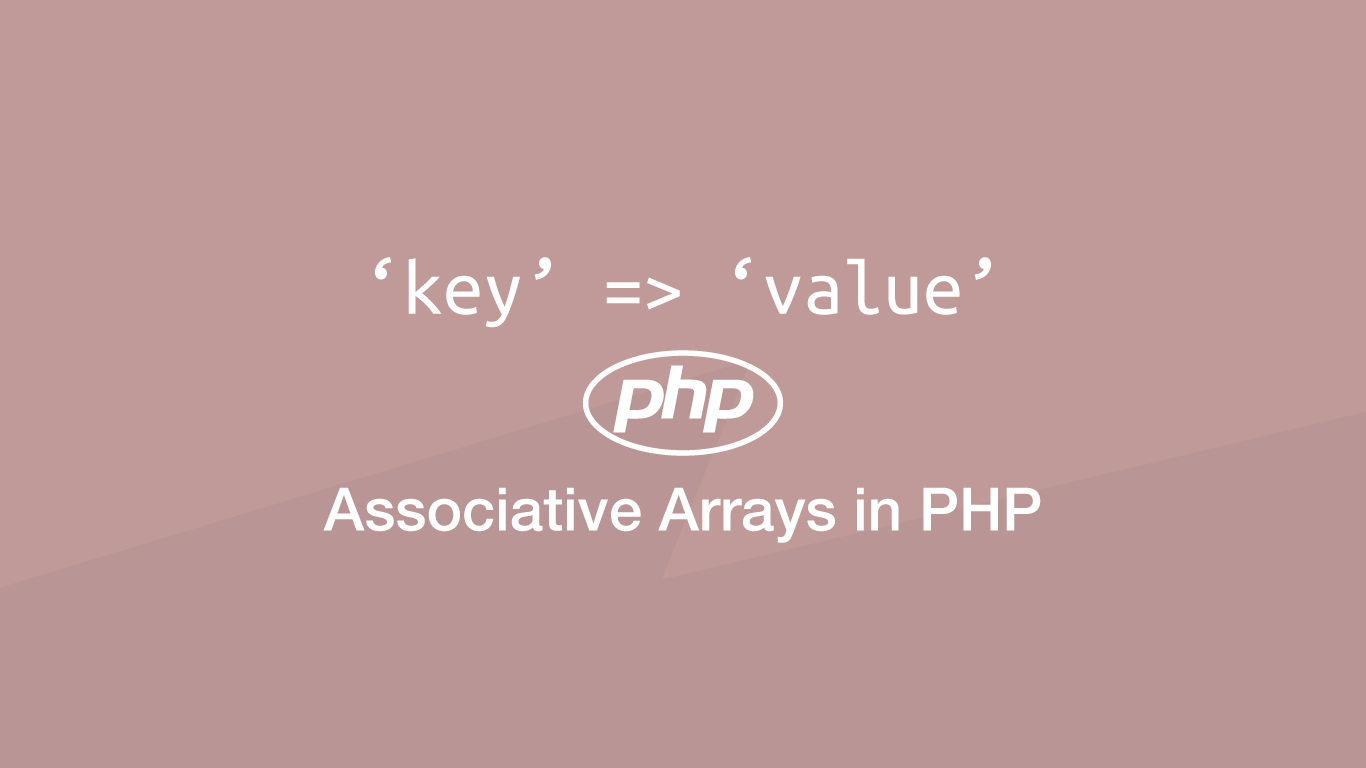# How to Use Associative Arrays in PHPAn associative array in PHP is just like any other array except its key names are associated with the values they contain rather than being numerical. Using associative arrays can aid the process of programming because it is easier to remember common key names than numbers.

In this tutorial will explore how to use associative arrays in PHP with examples.

## Creating an Associative Array

Let's begin by creating an associative array for a person. We can specify the key name and value between a `=>` (double arrow operator) and the indexes by a `,` (comma)

``````\$array = ['name' => 'John', 'location' => 'UK', 'age' => '28'];
``````

## Adding Elements to an Associative Array

To add an element to an associative array pass the name of the key inside `[]` (square brackets) followed by `=` (equals) and finally the value.

``````\$array['city'] = 'Manchester';
``````

## Changing the Value of an Element

Changing the value of an element in an associative array can be done in the exact same way as adding elements, though this time we would target an existing key name.

``````\$array['location'] = 'England';
``````

## Displaying an Associative Array

There are a couple of ways we could display an associative array. The most simple is to access and `echo` the value manually. In the example below, we are concatenating the values to display using a `.` (dot).

``````echo 'Name: ' . \$array['name'] . "<br>";
echo 'Location: ' . \$array['location'] . "<br>";
echo 'City: ' . \$array['city'] . "<br>";
echo 'Age: ' . \$array['age'] . "<br>";
``````
``````Name: John
Location: UK
City: Manchester
Age: 28
``````

This could be done in a cleaner way by using a `foreach`. In a `foreach` we can access the key names of the array dynamically as well as the values.

``````foreach (\$array as \$key => \$value) {
echo \$key .': '. \$value . "<br>";
}
``````
``````name: John
location: UK
age: 28
city: Manchester
``````

## Displaying Multidimensional Associative Arrays

Let's say we had an array containing associative arrays like this:

``````\$array = [
0 => ['name' => 'John', 'location' => 'UK', 'age' => '28'],
1 => ['name' => 'James', 'location' => 'UK', 'age' => '27']
];
``````

We could access the values on each associative array by nesting `[]` (square brackets) to the correct level.

``````foreach (\$array as \$key => \$value) {
echo \$array[\$key]['name'] . "<br>";
}
``````
``````John
James
``````

## Conclusion

You now know how to use associative arrays in PHP and when they might be more useful than using a numerical index.